Norton Theorem Norton theorem is just alternative of thevenin theorem . In Norton theorem , we just replace the circuit connected to...

## Norton Theorem

Norton theorem is just alternative of thevenin theorem. In Norton theorem, we just replace the circuit connected to a particular branch by equivalent current source. In this theorem, the circuit network is reduced into a single constant current source in which, the equivalent internal resistance is connected in parallel with it. Every voltage source can be converted into equivalent current source.
Suppose, in complex network we have to find out the current through a particular branch. If the network has one of more active sources, then it will supply current through the said branch. As in the said branch current comes from the network, it can be considered that the network itself is a current source. So in Norton theorem the network with different active sources is reduced to single current source that's internal resistance is nothing but the looking back resistance, connected in parallel to the derived source.

The looking back resistance of a network is the equivalent electrical resistance of the network when someone looks back into the network from the terminals where said branch is connected. During calculating this equivalent resistance, all sources are removed leaving their internal resistances in the network. Actually in Norton theorem, the branch of the network through which we have to find out the current, is removed from the network. After removing the branch, we short circuit the terminals where the said branch was connected. Then we calculate the short circuit current that flows between the terminals. This current is nothing but Norton equivalent current IN of the source. The equivalent resistance between the said terminals with all sources removed leaving their internal resistances in the circuit is calculated and said it is RN. Now we will form a current source that's current is IN A and internal shunt resistance is RN Ω.
For getting clearer concept of this theorem, we have explained it by the following example,
In the example two resistors R1 and R2 are connected in series and this series combination is connected across one voltage source of emf E with internal resistance Ri as shown. Series combination of one resistive branch of RL and another resistance R3 is connected across the resistance R2 as shown. Now we have to find out the current through RL by applying Norton theorem.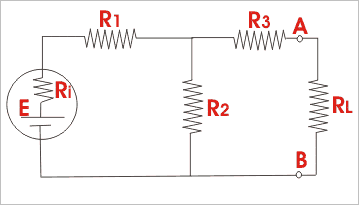First, we have to remove the resistor RL from terminals A and B and make the terminals A and B short circuited by zero resistance.
Second, we have to calculate the short circuit current or Norton equivalent current IN through the points A and B.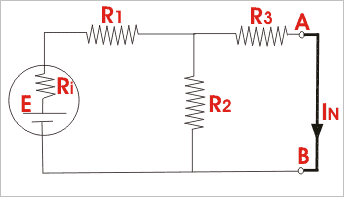The equivalent resistance of the network,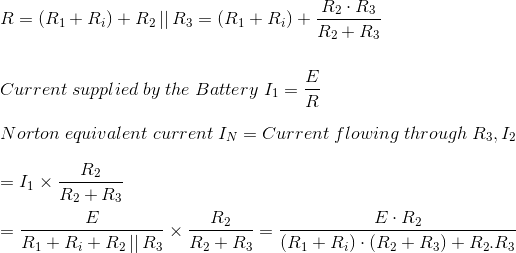To determine internal resistance or Norton equivalent resistance RN of the network under consideration, remove the branch between A and B and also replace the voltage source by its internal resistance. Now the equivalent resistance as viewed from open terminals A and B is RN,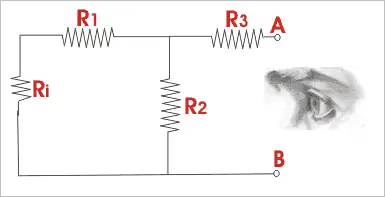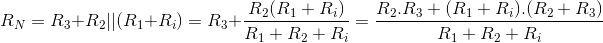As per Norton theorem, when resistance RL is reconnected across terminals A and B, the network behaves as a source of constant current IN with shunt connected internal resistance RN and this is Norton equivalent circuit.

## Norton Equivalent Circuit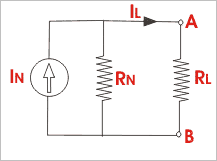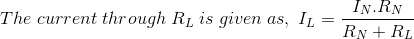Name

BASIC ELECTRICAL,12,BATTERIES,4,CIRCUIT THEORIES,9,CONTROL SYSTEMS,3,DC MOTOR,1,DIGITAL ELECTRONICS,1,DISTRIBUTED GENERATION,2,DISTRIBUTION,6,ELECTRICAL DRIVES,1,ELECTRICAL LAWS,8,ELECTRONICS DEVICES,2,General,7,GENERATION,3,GENERATOR,1,HIGH VOLTAGE,4,ILLUMINATION,1,INDUCTION MOTOR,7,MATERIALS,1,MEASUREMENT,1,MOTOR,1,POWER ELECTRONICS,2,PROJECTS ON INDUCTION MOTOR,1,PROTECTION,1,SMART GRID,3,SWITCHGEAR,4,SYNCHRONOUS MOTOR,1,TRANSFORMER,6,TRANSMISSION,4,
ltr
item
Electrical for Us: Norton Theorem | Norton Equivalent Current and Resistance
Norton Theorem | Norton Equivalent Current and Resistance